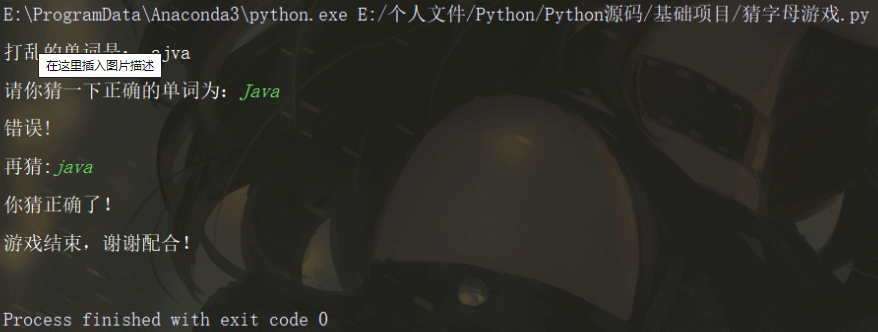﻿ 如何利用Python写猜数字和字母的游戏_python_脚本之家
python# 如何利用Python写猜数字和字母的游戏

## 猜数字游戏

```# -*- coding : utf-8 -*-
import random
num = random.randint(0, 100)
num1 = None
while num1 != num:
num1 = int(input("请再输入一次："))
if num1 > num:
print("你再猜小一点**************************")
elif num1 < num:
print("你再猜大一点**************************")
else:
print("真聪明*********************************")
break
print("恭喜你猜正确了")```## 猜字母游戏

```# -*- coding : utf-8 -*-
import random

word_1 = ["python", "linux", "mysql", "java", "hadoop", "pycharm", "smart"]
word = random.choice(word_1)
correct = word
jumble = ""
for _ in range(len(word)):
num = random.randrange(len(word))
jumble += word[num]
word = word[:num] + word[num + 1:]
print("打乱的单词是：", jumble)
guess = input("请你猜一下正确的单词为：")
while guess != correct or guess == "":
print("错误!")
guess = input("再猜:")
if guess == correct:
print("你猜正确了！")
print("游戏结束，谢谢配合！")```